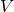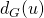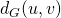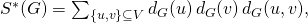Given a simple and connected graphwith vertex set, denoting by, the degree of a vertexand bythe distance of two vertices, the modified Schultz index ofis given bywhere the summation goes over all non ordered pairs of vertices of.
In this paper we consider some graph operations, namely cartesian product, complete product, composition and subdivision, and we obtain explicit formulae for the modified Schultz index of a graph in terms of the number of vertices and edges as well as some other topological invariants such as the Wiener index, the Schultz index and the first and second Zagreb indices.